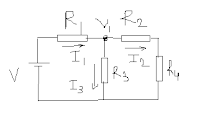### Circuit Analysis Problems and Solutions (Mesh and Nodal Analysis - Basic electrical electronics engineering, pdf)

Searching the books for your studies can be so easy
You can search in the search bar for your book .........................................................................

## Circuit Analysis Problems and solutions on Mesh and Nodal Analysis are provided below.

To discuss the concepts of basic circuit analysis, we are going to discuss here four circuits and the steps needed for circuit analysis. Circuit analysis problems in DC networks are basically of circuits which are made up of resistors and voltage source.

### Circuit Analysis Problems and Solutions 1:Circuit Analysis Problems and Solutions on mesh analysis
In this problem there are two loops. In the two loops, let two currents flow. By applying the concepts of mesh analysis we can solve out the value of current in the two loops. When you know the value of two loop currents, you can find out the voltage drops across the resistors present in the circuit.

Suppose it is said in question that resistor R4 is the load resistance and you need to find out the power consumed at load. When you know the current loop value, you can easily find the value of power consumed at load resistance R4. Because power is given by the product of current and voltage in resistor R4.

### Circuit Analysis Problems and Solutions 2:Circuit Analysis Problems and Solutions
In this second circuit analysis problem, there are three loops instead of two loops that we have discussed above. When three loops are present it means you are going to need three currents for the mesh analysis. Again you need to read my article on mesh analysis to learn the method of mesh analysis.

This network problem is more harder than the previous one.

### Circuit Analysis Problems and Solutions 3:Circuit analysis problems and solutions on nodal analysis
In this circuit analysis problems and solutions we are going to solve the problems using nodal analysis. To know how to electrical circuits or electronics circuits using nodal analysis, you need to read my article on nodal analysis method to solve the circuits.

In the above circuit there is only one node. The voltage across this node is V1. By using steps for nodal analysis, value of currents I (1,2,3 and 4) can be found easily.

## Circuit Analysis Problems and Solutions 4:Circuit Analysis Problems and solutions on nodal analysis
In this circuit analysis problem, we are given with two nodes in the circuit. By seeing that there are two nodes, we know two equations are formed. From these two equations value of voltages at nodes can be calculated. Two find the node equations, you need to know the steps for nodal analysis.

These are the some example circuit analysis problems and solutions hints provided to you. I hope you have got some basic idea about the circuit analysis problems. The area of circuit analysis is very vast and to begin with you must make your base strong.

For more readings on the circuit analysis, electrical theorems, you can read my articles. In each every theorem article given below, i have discussed a method to circuit analysis problem.